## 1 Introduction

Control dependencies between program statements are studied since 70’s. They have important applications in compiler optimizations [12, 14, 16], program analysis [9, 19, 36], and program transformations, especially program slicing [1, 9, 22, 26, 37]. Slicing is used in many areas including testing, debugging, parallelization, reverse engineering, program analysis and verification [17, 28].

Informally, two statements in a program are control dependent if one directly controls the execution of the other in some way. This is typically the case for if statements and their bodies. Control dependencies are nowadays classified as weak (non-termination insensitive) if they assume that a given program always terminates, or as strong (non-termination sensitive) if they do not have this assumption . We illustrate the difference on the control flow graph in Fig. 1. Node a controls whether b or c (and then d) is going to be executed, so b, c, and d are control dependent on a (the convention is to display dependence as edges in the “controls” direction). Similarly, b controls the execution of c and d, as these nodes may be bypassed by going from b to e. Note also that d controls whether d is going to be executed in the future and thus is control dependent on itself. However, c does not control d as any path from c hits d. All dependencies mentioned so far are weak, namely standard control dependencies as defined by Ferrante et al. . Weak control dependence assumes that the program always terminates, in particular, that the loop over d cannot iterate forever. As a result, e is reached by all executions and thus it is not weakly control dependent on any node. However, e is strongly control dependent on b and d. Indeed, if we assume that some executions can loop over d forever, then reaching e is controlled clearly by d and also by b as it can send the execution directly to e.

This paper is concerned with the computation of two prominent strong control dependencies introduced by Ranganath et al. [32, 33], namely non-termination sensitive control dependence (NTSCD) and decisive order dependence (DOD). NTSCD is studied in Sect. 3, which follows after preliminaries in Sect. 2. We first recall the definition of NTSCD and the algorithm of Ranganath et al.  for its computation. Then we show a flaw in the algorithm and suggest a fix. Finally, we introduce a new algorithm for the computation of NTSCD. Given a control flow graph with |V| nodes, the new algorithm runs in time $$O(|V|^2)$$, while the algorithm of Ranganath et al. runs in time $$O(|V|^4 \cdot \log |V|)$$ and its fixed version in time $$O(|V|^5)$$. We show a NTSCD relation of size $$\varTheta (|V|^2)$$, which means that our algorithm is asymptotically optimal.

The DOD relation captures the cases when one node controls the execution order of two other nodes. Roughly speaking, nodes $$\{b,c\}$$ are DOD on a whenever all executions passing through a eventually reach both b and c and a controls which is reached first. Ranganath et al.  proved that the relation is empty for reducible graphs , i.e., graphs where every cycle has a single entry point. Control flow graphs of structured programs are reducible, but irreducible graphs may arise for example in the following situations [11, 33, 35]:

• unstructured coding by a human, which is rather rare nowadays,

• compilation into unstructured code representation like JVM bytecode,

• tail call recursion optimization during compilation,

• when the control flow graph is interprocedural – in this case, irreducibility may be introduced by recursion or exceptions handling,

• by reversing a control flow graph containing, for example, break statements

• when the control flow graph is not generated from program, but, e.g., from a finite state machine.

The DOD relation is important (together with NTSCD) when we want to slice possibly non-terminating programs with irreducible control flow graphs and preserve their termination properties as well as data integrity [1, 33]. This is a common requirement when slicing is used as a preprocessing step before program verification [9, 23, 26], worst-case execution time analysis , information flow analysis [18, 19], analysis of concurrent programs  with busy-waiting synchronization or synchronization where possible spurious wake-ups of threads are guarded by loops (e.g., programs using the pthread library), and analysis of reactive systems and generic state-based models [2, 24, 33].

The DOD relation is studied in Sect. 4, where we recall its definition, discuss the Ranganath et al.’s algorithm for DOD , and show that this algorithm also contains a flaw. Fortunately, this flaw can be easily fixed without changing the complexity of the algorithm. Further, we develop a theory that underpins our new algorithm for the computation of DOD. Due to the space limitations, proofs of theorems can be found only in the extended version of this paper . The new algorithm, presented at the end of the section, computes DOD in time $$O(|V|^3)$$, while the original as well as the fixed version of the Ranganath et al.’s algorithm runs in $$O(|V|^5 \cdot \log |V|)$$. We show a DOD relation of size $$\varTheta (|V|^3)$$, which means that our algorithm is again asymptotically optimal.

Section 5 focuses on control closures (CC) introduced by Danicic et al. , which generalize control dependence to arbitrary directed graphs. It is known that the strong (i.e., non-termination sensitive) control closure for a set of nodes containing the starting node is equivalent to the closure under NTSCD and DOD relations. Hence, our algorithms for NTSCD and DOD can be used to compute strong CC in time $$O(|V|^3)$$ on control flow graphs, while the original algorithm by Danicic et al.  runs in $$O(|V|^4)$$.

Our theoretical contribution to computation of strong control dependencies is summarized in Table 1. Section 6 presents experimental evaluation showing that our algorithms are indeed dramatically faster than the original ones. The paper is concluded with Sect. 7.

### 1.1 Related Work

The first paper concerned with control dependence is due to Denning and Denning , who used control dependence to certify that flow of information in a program is secure. Weiser , Ottenstein and Ottenstein , and Ferrante et al.  used control dependence in program slicing, which is also the motivation for the most of the latter research in this area. These “classical” papers study control dependence in terminating programs with a unique exit node eventually reached by every execution. These restrictions have been gradually removed.

Podgurski and Clarke  defined the first strong control dependence that does not assume termination of the program.Footnote 1 However, their definitions and algorithms still require programs to have a unique exit node.

Bilardi and Pingal  introduced a framework that uses generalized dominance relation on graphs. In their framework, they are able to compute Podgurski and Clarke’s control dependence in $$O(|E|+|V|^2)$$ time for a directed graph (VE) with a unique exit node. In theory, NTSCD could be computed in their framework. However, computing augmented post-dominator tree – the central data structure of their framework – requires the unique exit node as it starts with post-dominator tree and, mainly, is much more complicated compared to our algorithm for NTSCD .

Chen and Rosu  introduced a parametric approach where loops can be annotated with information about termination. The resulting control dependence is somewhere between the classical and Podgurski and Clarke’s control dependence, the two being the extremes.

The notion of NTSCD and DOD was founded in works of Ranganath et al. [32, 33] in order to slice reactive systems, e.g., operating systems or controllers of embedded devices. They generalized also classical (non-termination insensitive) control dependence to graphs without the unique exit point (further investigated, e.g., by Androutsopoulos et al. ) and provided several relaxed versions of DOD.

Danicic et al.  introduced weak and strong control closures (CC) that generalize weak and strong control dependence (thus also NTSCD) to arbitrary graphs. They provide algorithms for the computation of minimal closures that run in $$O(|V|^3)$$ (weak CC) and $$O(|V|^4)$$ (strong CC) on graph with |V| nodes.

An orthogonal study of control dependence that arises between statements in different procedures (e.g., due to calls to exit()) was carried out by Loyall and Mathisen , Harrold et al. , and Sinha et al. .

## 2 Preliminaries

A finite directed graph is a pair $$G = (V,E)$$, where V is a finite set of nodes and $$E\subseteq V\times V$$ is a set of edges. If there is an edge $$(m,n)\in E$$, then n is called a successor of m, m is a predecessor of n, and the edge is an outgoing edge of m. Given a node n, $$Successors (n)$$ and $$Predecessors (n)$$ denote the sets of all its successors and predecessors, respectively. A path from a node $$n_1$$ is a nonempty finite or infinite sequence $$n_1n_2\ldots \in V^+\cup V^\omega$$ of nodes such that there is an edge $$(n_i,n_{i+1})\in E$$ for each pair $$n_i, n_{i+1}$$ of adjacent nodes in the sequence. A path is called maximal if it cannot be prolonged, i.e., it is infinite or the last node of the path has no outgoing edge. A node m is reachable from a node n if there exists a finite path such that its first node is n and its last node is m.

We say that a graph is a cycle, if it is isomorphic to a graph (VE) where $$V=\{n_1,\ldots ,n_k\}$$ for some $$k>0$$ and $$E=\{(n_1,n_2),(n_2,n_3),\ldots ,(n_{k-1},n_k),(n_k,n_1)\}$$. A cycle unfolding is a path in the cycle that contains each node precisely once.

In this paper, we consider programs represented by control flow graphs, where nodes correspond to program statements and edges model the flow of control between the statements. As control dependence reflects only the program structure, our definition of a control flow graph does not contain any statements. Our definition also does not contain any start or exit nodes as these are not important for the problems we study in this paper.

### Definition 1 (Control flow graph, CFG)

A control flow graph (CFG) is a finite directed graph $$G=(V,E)$$ where each node $$v\in V$$ has at most two outgoing edges. Nodes with exactly two outgoing edges are called predicate nodes or simply predicates. The set of all predicates of a CFG G is denoted by $$Predicates (G)$$.

## 3 Non-termination Sensitive Control Dependence

This section recalls the definition of NTSC by Ranganath et al.  and their algorithm for computing NTSCD. Then we show that the algorithm can produce incorrect results and introduce a new algorithm that is asymptotically faster.

### Definition 2

(Non-termination sensitive control dependence, NTSCD). Given a CFG $$G=(V,E)$$, a node $$n\in V$$ is non-termination sensitive control dependent (NTSCD) on a predicate node $$p\in Predicates (G)$$, written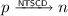, if p has two successors $$s_1$$ and $$s_2$$ such that

• all maximal paths from $$s_1$$ contain n, and

• there exists a maximal path from $$s_2$$ that does not contain n.

### 3.1 Algorithm of Ranganath et al.  for NTSCD

The algorithm is presented in Algorithm 1. Its central data structure is a two-dimensional array S where for each node n and for each predicate node p with successors r and s, S[np] always contains a subset of $$\{t_{pr},t_{ps}\}$$. Intuitively, $$t_{pr}$$ should be added to S[np] if n appears on all maximal paths from p that start with the prefix pr. The $$workbag$$ holds the set of nodes n for which some S[np] value has been changed and this change should be propagated. The first part of the algorithm initializes the array S with the information that each successor r of a predicate node p is on all maximal paths from p starting with pr. The main part of the algorithm then spreads the information about the reachability on all maximal paths in the forward manner. Finally, the last part computes the NTSCD relation according to Definition 2 and with use of the information in S.

The algorithm runs in time $$O(|E|\cdot |V|^3\cdot \log |V|)$$  for a CFG $$G=(V,E)$$. The $$\log |V|$$ factor comes from set operations. Since every node in CFG has at most 2 outgoing edges, we can simplify the complexity to $$O(|V|^4\cdot \log |V|)$$.

Although the correctness of the algorithm has been proved [32, Theorem 7], Fig. 2 presents an example where the algorithm provides an incorrect answer. The first part of the algorithm initializes S as shown in the figure and sets $$workbag$$ to $$\{2,6,3,4\}$$. Then any node from $$workbag$$ can be popped and processed. Let us apply the policy used for queues: always pop the oldest element in $$workbag$$. Hence, we pop 2 and nothing happens as the condition on line 17 is not satisfied for any m. This also means that the symbol $$t_{12}$$ is not propagated any further. Next we pop 6, which has no effect as 6 has no successor. By processing 3 and 4, $$t_{23}$$ and $$t_{24}$$ are propagated to S[5, 2] and 5 is added to the $$workbag$$. Finally, we process 5 and set S[6, 2] to $$\{t_{23},t_{24}\}$$. The final content of S is provided in the figure. Unfortunately, the information in S is sound but incomplete. In other words, if $$t_{pr}\in S[n,p]$$, then n is indeed on all maximal paths from p starting with pr, but the opposite implication does not hold. In particular, $$t_{12}$$ is missing in S[5, 1] and S[6, 1]. Consequently, the last part of the algorithm computes an incorrect NTSCD relation: it correctly identifies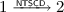,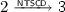, and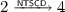, but it also incorrectly produces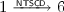and misses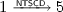.

A necessary condition to get the correct result is to process 2 only after 3, 4 are processed and $$S[5,6]=\{t_{23},t_{24}\}$$. For example, one obtains the correct S (also shown in the figure) when the nodes are processed in the order 3, 4, 2, 5, 6.

The algorithm is clearly sensitive to the order of popping nodes from $$workbag$$. We are currently not sure whether for each CFG there exists an order that leads to the correct result. An easy way to fix the algorithm is to ignore the $$workbag$$ and repeatedly execute the body of the while loop (lines 10–21) for all $$n\in V$$ until the array S reaches a fixpoint. However, this modification would slow down the algorithm substantially. Computing the fixpoint needs $$O(|V|^3)$$ iterations over the loop body (lines 10–21 excluding lines 14 and 21 handling the $$workbag$$) and one iteration of this loop body needs $$O(|V|^2)$$. Hence, the overall time complexity of the fixed version is $$O(|V|^5)$$.

### 3.2 New Algorithm for NTSCD

We have designed and implemented a new algorithm computing NTSCD. Our algorithm is correct, significantly simpler and asymptotically faster than the original algorithm of Ranganath et al. .

The new algorithm calls for each node n a procedure that identifies all NTSCD dependencies of n on predicate nodes. The procedure works in the following steps.

1. 1.

Color n red.

2. 2.

Pick an uncolored node such that it has some successors and they all are red. Color the node red. Repeat this step until no such node exists.

3. 3.

For each predicate node p that has a red successor and an uncolored one, output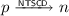.

Unlike the Ranganath et al.’s algorithm which works in a forward manner, our algorithm spreads the information about the reachability of n on all maximal paths in the backward direction starting from n.

The algorithm is presented in Algorithm 2. The procedure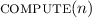implements the first two steps mentioned above. In the second step, it does not search over all nodes to pick the next node to color. Instead, it maintains the count of uncolored successors for each node. Once the count drops to 0 for a node, the node is colored red and the search continues with predecessors of this node. The third step is implemented directly in the main loop of the algorithm.

To prove that the algorithm is correct, we basically need to show that whenfinishes, a node m is red iff all maximal paths from m contain n. We start with a simple observation.

### Lemma 1

After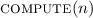finishes, a node m is red if and only if $$m=n$$ or m has a positive number of successors and all of them are red.

### Proof

For each node m, the counter is initialized to the number of its successors and it is decreased by calls to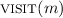each time a successor of m gets red. When the counter drops to 0 (i.e., all successors of the node are red), the node is colored red. Therefore, if m is red, it got red either on line 12 and $$m = n$$, or $$m\ne n$$ and m is red because all its successors got red (it must have a positive number of successors, otherwise the counter could not be 0 after its decrement). In the other direction, if $$m = n$$, it gets red on line 12. If it has a positive number of successors which all get red, the node is colored red by the argument above.   $$\square$$

### Theorem 1

Afterfinishes, for each node m it holds that m is red if and only if all maximal paths from m contain n.

### Proof

(“$$\Longleftarrow$$”) We prove this implication by contraposition. Assume that m is an uncolored node. Lemma 1 implies that each uncolored node has an uncolored successor (if it has any). Hence, we can construct a maximal path from m containing only uncolored nodes simply by always going to an uncolored successor, either up to infinity or up to a node with no successors. This uncolored maximal path cannot contain n which is red.

(“$$\Longrightarrow$$”) For the sake of contradiction, assume that there is a red node m and a maximal path from m that does not contain n. Lemma 1 implies that all nodes on this path are red. If the maximal path is finite, it has to end with a node without any successor. Lemma 1 says that such a node can be red if and only if it is n, which is a contradiction. If the maximal path is infinite, it must contain a cycle since the graph is finite. Let r be the node on this cycle that has been colored red as the first one. Let s be the successor of r on the cycle. Recall that $$r\ne n$$ as the maximal path does not contain n. Hence, node r could be colored red only when all its successors including s were already red. This contradicts the fact that r was colored red as the first node on the cycle.   $$\square$$

To determine the complexity of our algorithm on a CFG (VE), we first analyze the complexity of one run of. The lines 9–11 iterate over all nodes. The crucial observation is that the procedure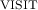is called at most once for each edge $$(m,m')\in E$$ of the graph: to decrease the counter of m when $$m'$$ gets red. Hence, the procedureruns in $$O(|V|+|E|)$$. This procedure is called on line 18 for each node n. Finally, lines 20–21 are executed for each pair of node n and predicate node p. This gives us the overall complexity $$O((|V|+|E|)\cdot |V| + |V|^2)=O((|V|+|E|)\cdot |V|)$$. Since in control flow graphs it holds $$|E|\le 2|V|$$, the complexity can be simplified to $$O(|V|^2)$$.

Note that our algorithm is asymptotically optimal as there are CFGs with NTSCD relations of size $$\varTheta (|V|^2)$$. For example, the CFG in Fig. 3 has $$|V|=2k+1$$ nodes and the corresponding NTSCD relation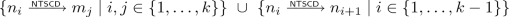is of size $$k^2+k-1\in \varTheta (|V|^2)$$.

## 4 Decisive Order Dependence

There are control dependencies not captured by NTSCD. For example, consider the CFG in Fig. 4. Nodes a and b are not NTSCD on p as they lie on all maximal paths from p. However, p controls which of a and b is executed first. Ranganath et al.  introduced the DOD relation to capture such dependencies.

### Definition 3 (Decisive order dependence, DOD)

Let $$G=(V,E)$$ be a CFG and $$p,a,b\in V$$ be three distinct nodes such that p is a predicate node with successors $$s_1$$ and $$s_2$$. Nodes ab are decisive order-dependent (DOD) on p, written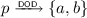, if

• all maximal paths from p contain both a and b,

• all maximal paths from $$s_1$$ contain a before any occurrence of b, and

• all maximal paths from $$s_2$$ contain b before any occurrence of a.

The importance of DOD for slicing of irreducible programs is discussed in the introduction.

### 4.1 Algorithm of Ranganath et al.  for DOD

Ranganath et al. provided an algorithm that computes the DOD relation for a given CFG $$G=(V,E)$$ in time $$O(|V|^4\cdot |E|\cdot \log |V|)$$ which amounts to $$O(|V|^5\cdot \log |V|)$$ on CFGs [33, Fig. 7]. The algorithm contains one unclear point. For each triple of nodes $$p,a,b\in V$$ such that $$p \in Predicates (G)$$ and $$a \ne b$$, the algorithm executes the following check and if it succeeds, then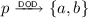is reported:

\begin{aligned} \textsc {reachable}(a,b,G) \wedge \textsc {reachable}(b,a,G) \wedge \textsc {dependence}(p,a,b,G) \end{aligned}
(1)

The procedure $$\textsc {dependence} (p,a,b,G)$$ returns true iff a is on all maximal paths from one successor of p before any occurrence of b and b is on all maximal paths from the other successor of p before any occurrence of a. The procedure $$\textsc {reachable}$$ is specified only by words [33, description of Fig. 7] as follows:

$$\textsc {reachable}(a,b,G)$$ returns true if b is reachable from a in the graph G.

Unfortunately, this algorithm can provide incorrect results. For example, consider the CFG in Fig. 5. Nodes pab satisfy the formula (1): a appears on all maximal paths from one successor of p (namely a) before any occurrence of b, and b appears on all maximal paths from the other successor of p (which is b) before any occurrence of a. At the same time, a and b are reachable from each other. However, it is not true that, because a and b do not lie on all maximal paths from p (the first condition of Definition 3 is violated).

The algorithm can be fixed by modifying the procedure $$\textsc {reachable} (a,b,G)$$ to return true if b is on all maximal paths from a. The modified procedure can be implemented with use of the procedure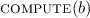of Algorithm 2. As the procedure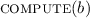runs in $$O(|V|+|E|)$$, the modification does not increase the overall complexity of the algorithm. By comparing the fixed and the original version of $$\textsc {reachable} (a,b,G)$$, one can readily confirm that the original version produces supersets of DOD relations.

### 4.2 New Algorithm for DOD: Crucial Observations

As in the case of NTSCD, we have designed a new algorithm for the computation of DOD, which is relatively simple and asymptotically faster than the DOD algorithm of Ranganath et al. .

Given a CFG, our algorithm first computes for each predicate p the set $$V_p$$ of nodes that are on all maximal paths from p. The definition of DOD implies that only pairs of nodes in $$V_p$$ can be DOD on p. For every predicate p we build an auxiliary graph $$A_p$$ with nodes $$V_p$$ and from this graph we get all pairs of nodes that are DOD on p. The graph $$A_p$$ is defined as follows.

### Definition 4

($$V'\!$$-interval ). Given a CFG $$G=(V,E)$$ and a subset $$V' \subseteq V$$, a path $$n_1 \dots n_k$$ such that $$k \ge 2$$, $$n_1,n_k\in V'$$, and $$\forall 1< i < k: n_i\not \in V'$$ is called a $$V'\!$$-interval from $$n_1$$ to $$n_k$$ in G.

In other words, a $$V'\!$$-interval is a finite path with at least one edge that has the first and the last node in $$V'$$ but no other node on the path is in $$V'$$.

### Definition 5

(Graph $$A_p$$Footnote 2). Given a CFG $$G=(V,E)$$, a predicate node $$p \in Predicates (G)$$ and the subset $$V_p \subseteq V$$ of nodes that are on all maximal paths from p, the $$A_p=(V_p, E_p)$$ is the graph where

$$E_p = \{(x,y)\mid \text {there exists a} \; V_p\! \; \text {-interval from} \; x \; \text {to} \; y \; \text {in} \; G \}.$$

In this subsection, we describe the connections between these graphs and DOD that underpin our algorithm. The proofs of the theorems can be found in the extended version of this paper .

Given a predicate p of a CFG G, the graph $$A_p$$ does not have to be a CFG as nodes in $$A_p$$ can have more than two successors. However, $$A_p$$ preserves exactly all possible orders of the first occurrences of nodes in $$V_p$$ on maximal paths in G starting from p. More precisely, for each maximal path from p in G, there exists a maximal path from p in $$A_p$$ with the same order of the first occurrences of all nodes in $$V_p$$, and vice versa. Further, it turns out that there are no nodes DOD on p unless $$A_p$$ has the right shape.

### Definition 6

(Right shape of $$A_p$$ ). Given a CFG G, a predicate node $$p \in Predicates (G)$$ and the graph $$A_p=(V_p, E_p)$$, we say that $$A_p$$ has the right shape if it consists only of a cycle and the node p with at least two edges going to some nodes on the cycle (i.e., the nodes of $$V_p\smallsetminus \{p\}$$ can be labeled $$n_1,\ldots ,n_k$$ such that $$E_p=\{(n_1,n_2),(n_2,n_3),\ldots ,(n_{k-1},n_k),(n_k,n_1)\}\cup \{(p,n_i)\mid i\in I\}$$ for some $$I\subseteq \{1,\ldots ,k\}$$ with $$|I|\ge 2$$).

Figure 6 depicts an $$A_p$$ which has the right shape. In the following text, we work only with $$A_p$$ graphs in the right shape.

Let $$s_1$$ and $$s_2$$ be the two successors of p in G. Note that $$s_1$$ and $$s_2$$ may, but do not have to be in $$A_p$$. To compute the pairs of nodes that are DOD on p, we need to know all possible orders of the first occurrences of nodes in $$V_p$$ on the maximal paths in G starting in $$s_1$$ and $$s_2$$. Hence, for each successor $$s_i$$ we compute the set $$S_i$$ of nodes that appear as the first node of $$V_p$$ on some maximal path from $$s_i$$ in G. Formally, for $$i\in \{1,2\}$$, we define

$$S_i=\{n\in V_p\mid \text {there exists a path} \; s_i\ldots n\in (V\smallsetminus V_p)^*.V_p \; \text {in} \; G \}.$$

The nodes in $$S_1\cup S_2$$ are exactly all the successors of p in $$A_p$$. Further, the maximal paths from the nodes of $$S_i$$ in $$A_p$$ reflect exactly all possible orders of the first occurrences of nodes in $$V_p$$ on maximal paths in G starting in $$s_i$$. If $$S_1$$ and $$S_2$$ are not disjoint, then there exist two maximal paths in G, one starting in $$s_1$$ and the other in $$s_2$$, that differ only in prefixes of nodes outside $$V_p$$. The definition of DOD implies that there are no nodes DOD on p in this case. Therefore we assume that $$S_1$$ and $$S_2$$ are disjoint.

The nodes in $$S_i$$ divide the cycle of $$A_p$$ into $$s_i$$-strips, which are parts of the cycle starting with a node from $$S_i$$ and ending before the next node of $$S_i$$.

### Definition 7

($$s_i$$-strip). Let $$i\in \{1,2\}$$. An $$s_i$$-strip is a path $$n\ldots m\in S_i.(V_p\smallsetminus S_i)^*$$ in $$A_p$$ such that the successor of m in $$A_p$$ is a node in $$S_i$$.

An example of $$A_p$$ with $$s_i$$-strips is in Fig. 6. The $$s_i$$-strips directly say which pairs of nodes of $$V_p$$ are in the same order on all maximal paths from $$s_i$$ in G. In particular, a node a is before any occurrence of node b on all maximal paths from a successor s of p in G if and only if there is an s-strip containing both a and b where a is before b. As a corollary, we get the following theorem:

### Theorem 2

Let p be a predicate with successors $$s_1,s_2$$ such that $$A_p$$ has the right shape and $$S_1\cap S_2=\emptyset$$. Then nodes $$a,b\in V_p$$ are DOD on p if and only if

• there exists an $$s_1$$-strip in $$A_p$$ that contains a before b and

• there exists an $$s_2$$-strip in $$A_p$$ that contains b before a.

Consider again the $$A_p$$ in Fig. 6. The theorem implies that nodes $$n_1,n_5$$ are DOD on p as they appear in $$s_1$$-strip $$n_1n_2n_3n_4n_5n_6$$ and in $$s_2$$-strip $$n_5n_6n_7n_8n_1$$ in the opposite order. Nodes $$n_1,n_6$$ are DOD on p for the same reason.

With use of the previous theorem, we can find a regular language over $$V_p$$ such that there exist nodes ab DOD on p iff some unfolding of the cycle in $$A_p$$ is in the language.

### Theorem 3

Let p be a predicate with successors $$s_1,s_2$$ such that $$A_p$$ has the right shape and $$S_1\cap S_2=\emptyset$$. Further, let $$U=V_p\smallsetminus (S_1\cup S_2)$$. There are some nodes ab DOD on p if and only if the cycle in $$A_p$$ has an unfolding of the form $$S_1.U^*.(S_2.U^*)^*.S_2.U^*.(S_1.U^*)^*$$.

Finally, an unfolding of the mentioned form can be directly used for the computation of nodes that are DOD on p.

### Theorem 4

Let p be a predicate with successors $$s_1,s_2$$ such that $$A_p$$ has the right shape and $$S_1\cap S_2=\emptyset$$. Further, let $$A_p$$ have an unfolding of the form $$S_1.U^*.(S_2.U^*)^*.S_2.U^*.(S_1.U^*)^*$$ where $$U=V_p\smallsetminus (S_1\cup S_2)$$. Then there is exactly one path $$m_1\ldots m_i\in S_1.U^*.S_2$$ and exactly one path $$o_1\ldots o_j\in S_2.U^*.S_1$$ on the cycle. Moreover,if and only if $$m_1\ldots m_{i-1}$$ contains a and $$o_1\ldots o_{j-1}$$ contains b (or the other way round).

### 4.3 New Algorithm for DOD: Pseudocode and Complexity

Our DOD algorithm is shown in Algorithms 3 and 4. As nearly all applications of DOD need also NTSCD, we present the algorithm with a simple extension (gray lines with asterisks) that simultaneously computes NTSCD.

The DOD algorithm starts at line 20 of Algorithm 4. The first step is to compute the sets $$V_p$$ for all predicate nodes p of a given CFG G. The computation of predicate nodes can be found in Algorithm 3. It is a slightly modified version of Algorithm 2. Recall that the procedureof Algorithm 2 marks red every node such that all maximal paths from the node contain n. The procedureof Algorithm 3 does in principle the same, but instead of the red color it marks the nodes with the identifier of the node n. Every node m collects these marks in set $$V_m$$. After we runfor all the nodes n in the graph, each node m has in its set $$V_m$$ precisely all nodes that are on all maximal paths from m. For the computation of DOD, only the sets $$V_p$$ for predicate nodes p are needed, but the extension computing NTSCD may use all these sets.

When the sets $$V_p$$ are calculated, we compute DOD (and NTSCD) dependencies for each predicate node separately by procedures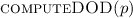and computeNTSCD(p). The procedure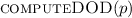first constructs the graph $$A_p$$ with the use of build$$A_p(p)$$. Nodes of the graph are these of $$V_p$$. To compute edges, we trigger depth-first search in G from each $$n\in V_p$$. If we find a node $$m \in V_p$$, we add the edge (nm) to the graph $$A_p$$ and stop the search on this path. When the graph $$A_p$$ is constructed, we check whether it has the right shape. If not, we return $$\emptyset$$ as there are no nodes DOD on p in this case.

The next step is to compute the sets $$S_1$$ and $$S_2$$. Again, we apply a similar depth-first search as in the construction of $$A_p$$ described above. If the sets $$S_1,S_2$$ are not disjoint, we return $$\emptyset$$ as there are no nodes DOD on p.

Then we unfold the cycle in $$A_p$$ from an arbitrary node in $$S_1$$, compute the set U, and check whether the unfolding matches $$(S_1.U^*)^+.(S_2.U^*)^+.(S_1.U^*)^*$$. Note that any unfolding starting in $$S_1$$ matches this language iff the cycle has an unfolding of the form $$S_1.U^*.(S_2.U^*)^*.S_2.U^*.(S_1.U^*)^*$$ of Theorem 3. Hence, we return $$\emptyset$$ if the check fails.

Finally, we extract the paths of the form $$S_1.U^*.S_2$$ and $$S_2.U^*.S_1$$ from the unfolding. Note that the last node of the latter path can be the first node of the unfolding. Finally, we compute the DOD dependencies according to Theorem 4.

The procedure computeNTSCD(p) used for the computation of NTSCD simply follows Definition 2: it makes dependent on p each node that is on all maximal paths from the successor $$s_1$$ but not on all maximal paths from the successor $$s_2$$ or symmetrically for $$s_2$$ and $$s_1$$.

As the correctness of our algorithm comes directly from the observations made in the previous subsection, it remains only to analyze its complexity. The procedure compute$$V_p$$ s consists of two cycles in sequence. The first cycle runs in O(|V|). The second cycle calls O(|V|)-times the procedure. This procedure is essentially identical to the procedure of the same name in Algorithm 2 and so is its time complexity, namely $$O(|V|+|E|)$$. Note that sets can be represented by bitvectors and therefore adding an element and checking the presence of an element in a set are constant-time. Overall, the procedure compute$$V_p$$ s runs in $$O(|V|\cdot (|V|+|E|))$$, which is $$O(|V|^2)$$ for CFGs.

Now we discuss the complexity of the procedure computeDOD(p). Creating the graph $$A_p$$ requires calling depth-first search O(|V|) times, which yields $$O(|V|\cdot |E|)$$ in total. Computation of $$S_1,S_2$$ requires another two calls of depth-first search, which is in O(|E|). When sets are represented as bitvectors, checking that $$S_1$$ and $$S_2$$ are disjoint is in O(|V|). Unfolding the cycle, matching the unfolding to the language (line 10), and the procedure extract run also in O(|V|). The construction of the DOD relation on line 14 is in $$O(|V|^2)$$. Altogether, computeDOD(p) runs in $$O(|V|\cdot |E| + |V|^2)$$ which simplifies to $$O(|V|^2)$$ for CFGs.

computeDOD is called O(|V|) times, so the overall complexity of computing DOD for a CFG $$G=(V,E)$$ is $$O(|V|^3)$$. If we compute also NTSCD, we make O(|V|) extra calls to computeNTSCD(p), where one call takes O(|V|) time. Therefore, the asymptotic complexity of computing NTSCD with DOD does not change from computing DOD only.

Our algorithm running in time $$O(|V|^3)$$ is asymptotically optimal as there exist graphs with DOD relations of size $$\varTheta (|V|^3)$$. For example, the CFG in Fig. 7 has $$|V|=4k+1$$ nodes and the corresponding DOD relation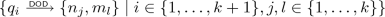is of size $$k^3+k^2\in \varTheta (|V|^3)$$.

## 5 Comparison to Control Closures

In 2011, Danicic et al.  introduced control closures (CC) that generalize control dependence from CFGs to arbitrary graphs. In particular, strong control closure, which is sensitive to non-termination, generalizes strong control dependence including NTSCD and DOD.

### Definition 8 (Strongly control-closed set)

Let $$G = (V, E)$$ be a CFG and let $$U\subseteq V$$. The set U is strongly control-closedFootnote 3 in G if and only if for every node $$v\in V \smallsetminus U$$ that is reachable in G from a node in U, one of these holds:

• there is no node in U reachable from v or

• there exists a node $$u\in U$$ such that all maximal paths from v contain u and it is the first node from U on all these paths.

In other words, whenever we leave a strongly control-closed set, we either cannot return back or we have to return back to the set in a certain node.

### Definition 9 (Strong control closure, strong CC)

Let $$G = (V, E)$$ be a CFG and $$V'\subseteq V$$. A strong control closure (strong CC) of $$V'$$ is a strongly control-closed set $$U\supseteq V'$$ such that there is no strongly control-closed set $$U'$$ satisfying $$U\supsetneq U'\supseteq V'$$.

Danicic et al. present an algorithm for the computation of strong control closures running in $$O(|V|^4)$$ [13, Theorem 66]. In fact, the algorithm uses a procedure $$\varGamma$$ that is very similar to our procedureof Algorithm 2.

We can also define the closure of a set of nodes under NTSCD and DOD.

### Definition 10 (NTSCD and DOD closure)

Let $$G = (V, E)$$ be a CFG. A NTSCD and DOD closure of a set $$V'\subseteq V$$ is the smallest set $$U\supseteq V'$$ satisfying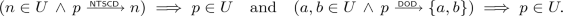Definition 10 directly provides an algorithm computing the NTSCD and DOD closure of a given set $$V'\subseteq V$$. Roughly speaking, if we represent the NTSCD relation with edges and the DOD relation with hyperedges in a directed hypergraph with nodes V, the closure computation amounts to gathering backward reachable nodes from $$V'$$.

Danicic et al. [13, Lemmas 93 and 94] proved that for a CFG $$G = (V,E)$$ with a distinguished $$start$$ node from which all nodes in V are reachable and a subset $$U\subseteq V$$ such that $$start \in U$$, the set U is strongly control-closed iff it is closed under NTSCD and DOD. Hence, on graphs with such a $$start$$ node, the strong CC of a set $$V'$$ containing the $$start$$ node can be computed also by computing its NTSCD and DOD closure. Computation of the NTSCD and DOD closure runs in $$O(|V|^3)$$ as the backward reachability is dominated by the computation of NTSCD and DOD relations.

A substantial difference between the algorithm for strong CC by Danicic et al.  and our algorithm is that we are able to compute DOD and NTSCD separately, whereas the former is not. Moreover, our algorithm for NTSCD and DOD closure is asymptotically faster.

## 6 Experimental Evaluation

We implemented our algorithms for the computation of NTSCD, DOD, and the NTSCD and DOD closure in C++ on top of the LLVM  infrastructure. The implementation is a part of the library for program analysis and slicing called DG , which is used for example in the verification and test generation tool Symbiotic . We also implemented the original Ranganath et al.’s algorithms for NTSCD and DOD, the fixed versions of these algorithms from Subsects. 3.1 and 4.1, and the algorithm for the computation of strong CC by Danicic et al.

In the implementation of the strong CC algorithm by Danicic et al. , we use our procedureof Algorithm 2 to implement the function $$\varGamma$$. This should have only a positive effect as this procedure is more efficient than iterating over all edges in a copy of the graph and removing them .

In our experiments, we use CFGs of functions (where nodes of the CFG represent basic blocks of the function) obtained in the following way. We took all benchmarks from the Competition on Software Verification (SV-COMP) 2020.Footnote 4 These benchmarks contain many artificial or generated code, but also a lot of real-life code, e.g., from the Linux project. Each source code file was compiled with clang into LLVM and preprocessed by the -lowerswitch pass to ensure that every basic block has at most two successors. Then we extracted individual functions and removed those with less than 100 basic blocks, as the computation of control dependence runs swiftly on small graphs. Because it is possible that one function is present in multiple benchmarks, the next step was to remove these duplicate functions. For every function, we computed the number of nodes and edges in its CFG, and performed DFS on the CFG to obtain the number of tree, forward, cross and back edges, and the depth of the DFS tree. If two or more functions shared the name and all the computed numbers, we kept only one such function. Note that this process may have removed also a function that was not a duplicate of some other, but only with a low probability. At the end, we were left with 2440 functions. The biggest function has 27851 basic blocks. Table 2 shows the distribution of the sizes of the generated CFGs.

The experiments were run on machines with AMD EPYC CPU with the frequency 3.1 GHz. Each benchmark run was constrained to 1 core and 8 GB of RAM. We used the tool Benchexec  to enforce resources isolation and to measure their usage. All presented times are CPU times. We set the timeout to 100 s for each algorithm run.

In the following, original algorithms refers to the algorithms of Ranganath et al. (we distinguish between the incorrect and the fixed versions when needed) and new algorithms refers to the algorithms introduced in this paper.

NTSCD Algorithms. In the first set of experiments, we compared the new algorithm for NTSCD against the incorrect and the fixed version of the original NTSCD algorithm. Although it seems that comparing to the incorrect version is meaningless, we did not want to compare only to the fixed version as the provided fix slows down the algorithm.

The results are depicted in Fig. 8. On the left scatter plot, there is the comparison of the new algorithm to the incorrect original algorithm and on the right scatter plot we compare to the fixed original algorithm. As we can see, the new algorithm outperforms the original algorithm significantly. The incorrect original algorithm produced a wrong NTSCD relation in 98.6 % of the considered benchmarks. The fixed version of the original algorithm returned precisely the same NTSCD relations as the new algorithm. We can also see that the scatter plot on the right contains more timeouts of the original algorithm. It supports the claim that the fix slows down the original algorithm.

DOD Algorithms. We compared the new DOD algorithm to the fixed version of the original DOD algorithm. As the fix does not change the asymptotic complexity of the original algorithm, we do not compare the new algorithm with the incorrect version of the original algorithm. The results of the experiments are displayed in Fig. 9 (left). We can see that the new algorithm is again very fast. In fact, the results resemble the results of the pure NTSCD algorithm, which is basically the part of the DOD algorithm that computes $$V_p$$ sets. It benefits from early checks that detect predicate nodes with no DOD dependencies.

As mentioned in the introduction, DOD is empty for structured programs as their CFGs are reducible. We do not know precisely how many of the 2440 considered functions have irreducible CFGs, but we know that 2373 of them use goto statements. DOD relations for 12 functions was non-empty, which means that CFGs of these functions are irreducible. Note that there may have been other irreducible CFGs with empty DOD relation.

Additionally, we tested the DOD algorithms on randomly generated graphs, where we can expect that irreducible graphs emerge more often. Figure 9 (right) shows the results for graphs that have 500 nodes and 50, 100, 150, ...randomly distributed edges (such that every node has at most two successors). Each presented running time is in fact an average of 10 measurements with different random graphs. We can see that the new algorithm is agnostic to the number of edges. Its running time in this experiment ranges from $$4.12\cdot 10^{-3}$$ to $$8.89\cdot 10^{-3}$$ seconds. The original DOD algorithm does not scale well with the increasing number of edges.

Strong CC Algorithm. We also compare the strong CC algorithm of Danicic et al.  against our NTSCD and DOD closure algorithm on sets of nodes containing a distinguished $$start$$ node, where these two algorithms produce equivalent results. For these experiments, we need a starting set that is going to be closed. We decided to run these experiments on the considered functions that have at least two exit points. The starting set consists of the node representing the entry point and the node representing one of the exit points. The closure of this set contains all nodes that may influence getting to the other exit points. The results are shown on the scatter plot in Fig. 10. Our algorithm clearly outperforms the strong CC algorithm.